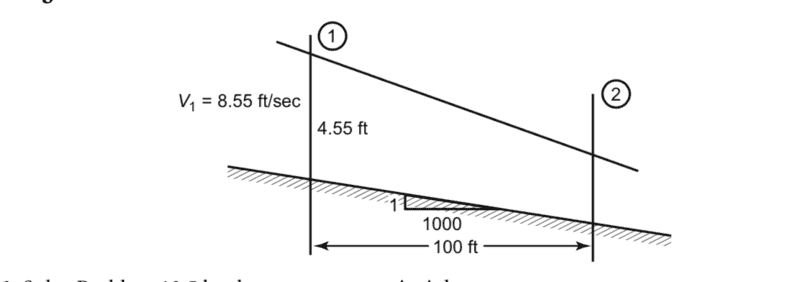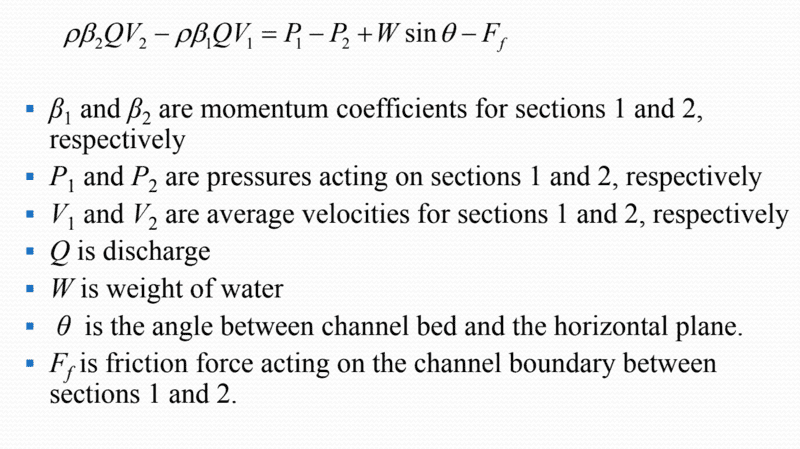# Momentum equation and open channel flow

## Homework Statement

Determine y2 (ft)and v2 (ft/s) using the momentum principle assume widths are the same at 1 and 2## Homework Equations

from what i remember in my past fluids class, the momentum equation is:
Force = Qvout - Qvin assuming same density and direction

However in my lecture the professor provided another equationbut when i work out the units in this equation left side yields Force and right side is pressure (force/area)

did my professor forget to multiply each pressure with its respective Area? P1A1?

## The Attempt at a Solution

using ##\rho####\beta##QV2 - ##\rho####\beta##QV1 = P1A1-P2A2
assume both ##\beta##'s = 1
assume width = 1
using continuity equation Q = V1A1 = V2A2 = (4.55)(1)(8.55)=y2(1)V2

##\rho##(4.55)(1)(8.55)(V2 - V1)= P1(4.55)(1)-P2y2(1)

##\rho##38.9(V2 - 8.55)= P14.55-P2y2

I am stuck

Chestermiller
Mentor

## Homework Statement

Determine y2 (ft)and v2 (ft/s) using the momentum principle assume widths are the same at 1 and 2
View attachment 230419

## Homework Equations

from what i remember in my past fluids class, the momentum equation is:
Force = Qvout - Qvin assuming same density and direction

However in my lecture the professor provided another equation

View attachment 230421
but when i work out the units in this equation left side yields Force and right side is pressure (force/area)

did my professor forget to multiply each pressure with its respective Area? P1A1?

## The Attempt at a Solution

using ##\rho####\beta##QV2 - ##\rho####\beta##QV1 = P1A1-P2A2
assume both ##\beta##'s = 1
assume width = 1
using continuity equation Q = V1A1 = V2A2 = (4.55)(1)(8.55)=y2(1)V2

##\rho##(4.55)(1)(8.55)(V2 - V1)= P1(4.55)(1)-P2y2(1)

##\rho##38.9(V2 - 8.55)= P14.55-P2y2

I am stuck
You are correct about needing to get the pressures times the areas, but the pressure is varying with depth, so you need to integrate the pressure (which is hydrostatic in the y direction) over the area. Also note that, from the continuity equation, y2 is a function of v2.

What are you doing about the friction term?

Solved it, my professor's notes are filled with errors as he calls P1,P2 a pressure when really it is a Force, also he has some typos on the equations. Where I was confused was, I thought (from my fluid dynamics class) when we look at a controlled volume we immediately add forces on the cut which where (Pressure) X (Cross sectional Area) where as here we take the integral of ##\rho##gydA from 0 to h where dA = (width)dy

What is the difference?

Chestermiller
Mentor
Solved it, my professor's notes are filled with errors as he calls P1,P2 a pressure when really it is a Force, also he has some typos on the equations. Where I was confused was, I thought (from my fluid dynamics class) when we look at a controlled volume we immediately add forces on the cut which where (Pressure) X (Cross sectional Area) where as here we take the integral of ##\rho##gydA from 0 to h where dA = (width)dy

What is the difference?
What is what difference?

last semester, in a different course when we analyzed a controlled volume the pressure force was simply P X (Cross sectional Area).

Now we integrate with respect to depth geometry

Was it for simplicity's sake that we did P X A?

Chestermiller
Mentor
In the cases you looked at, the channel was closed, and there was no free surface, and the average pressure was much higher than the hydrostatic difference in pressure between the top of the channel and the bottom of the channel. Here, the pressure is zero at the top, and twice the average at the bottom.

True, thank you for your help, you a real one.